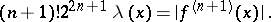# Local approximation of functions

Jump to: navigation, search

A measure of approximation (in particular, best approximation) of a functionon a set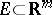, regarded as a function of this set. The main interest is in the behaviour of a local approximation of a function as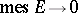. In certain cases it is possible to characterize the degree of smoothness of the function to be approximated in terms of a local approximation of the function. Let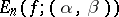be the best approximation of a function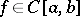by algebraic polynomials of degreeon an interval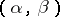,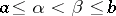. The following assertion holds: A necessary and sufficient condition for a functionto have a continuous derivative of orderat all points ofis thatuniformly for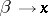,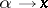,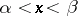, where the continuous functionis defined by